Half equations to overall equations The two half reactions are given below. Identify the oxidation and reduction half reactions and give the overall equation. Print this sheet out and complete the exercise. Or Copy this document into Word Copper metal is placed in a solution of silver nitrate.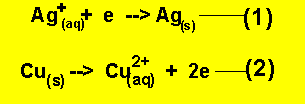Oxidation is ___ 1 2 Reduction is ___ 1 2 Overall equation Zinc metal is placed in an acid solution.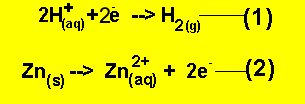Oxidation is ___ 1 2 Reduction is ___ 1 2 Overall equation Aluminium metal reacts with oxygen gas to produce aluminium oxide.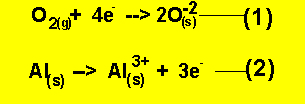Oxidation is ___ 1 2 Reduction is ___ 1 2 Overall equation Iron rusts in water according to the half equations below.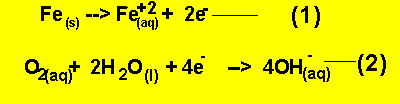Oxidation is ___ 1 2 Reduction is ___ 1 2 Overall equation Iron 2+ ion is converted to iron 3+ by the permanganate ion according to the half equations below.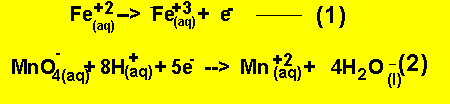Oxidation is ___ 1 2 Reduction is ___ 1 2 Overall equation Continue with overall to half equations.

Home